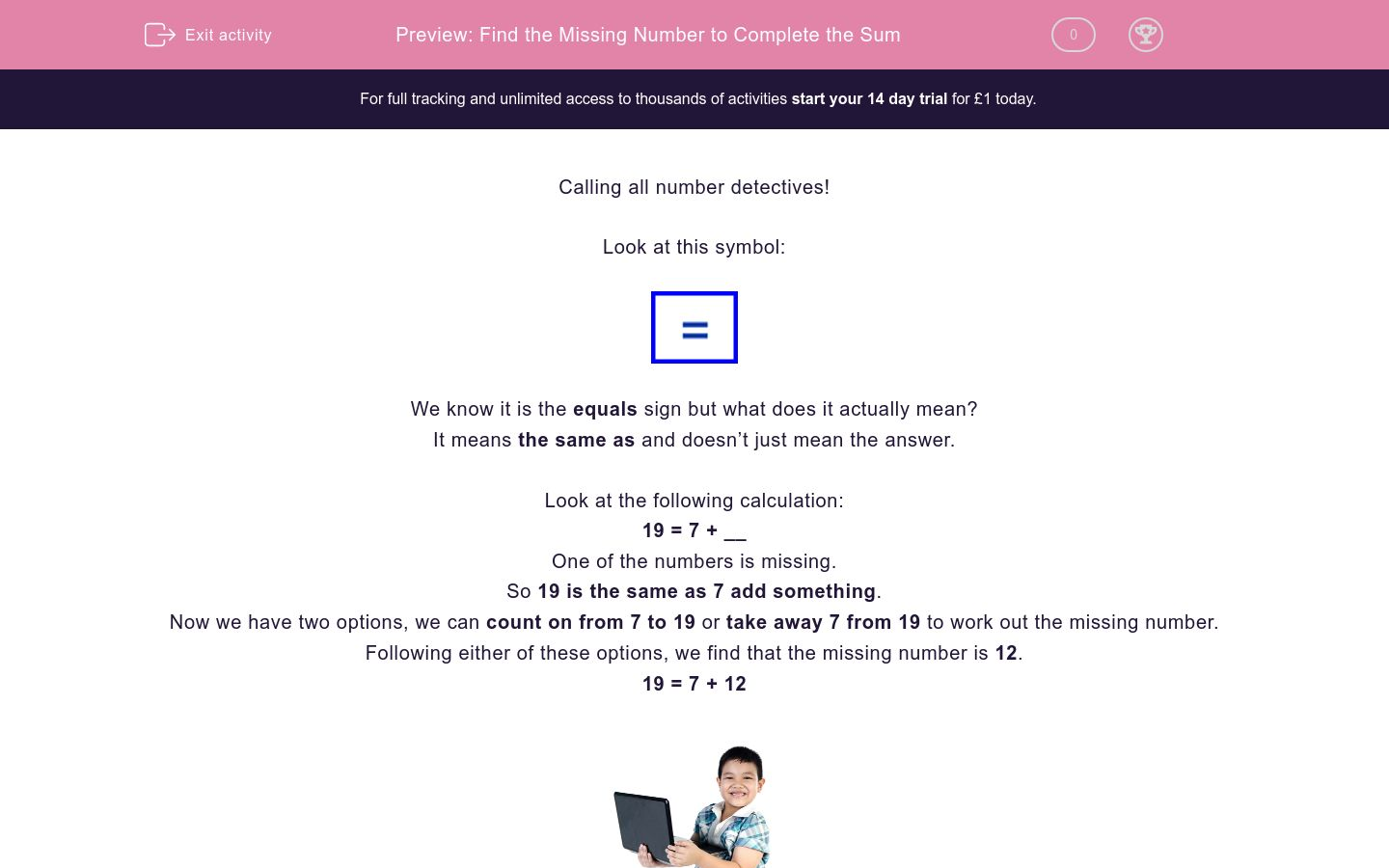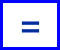# Find the Missing Number to Complete the Sum

In this worksheet, students will complete a calculation by adding in the missing numbers. It will strengthen their number skills.Key stage:  KS 2

Curriculum topic:   Verbal Reasoning

Curriculum subtopic:   Complete the Calculation

Difficulty level:### QUESTION 1 of 10

Calling all number detectives!

Look at this symbol:We know it is the equals sign but what does it actually mean?

It means the same as and doesn’t just mean the answer.

Look at the following calculation:

19 = 7 + __

One of the numbers is missing.

So 19 is the same as 7 add something.

Now we have two options, we can count on from 7 to 19 or take away 7 from 19 to work out the missing number.

Following either of these options, we find that the missing number is 12.

19 = 7 + 12Let’s try another:

17 = 20 - __

In this question, the answer of 17 is the same as 20 take away something.

What do we need to take away from 20 to reach 17?

So the missing number is 3.

17 = 20 - 3

Remember that equals means the same as and the answers on either side of the equals sign must be the same.

In this activity, you will be completing sums by finding the missing number.

Good luck!

Are you ready to start an exciting number activity?

Below is a calculation. One of the numbers has been taken away by the cute little puppy below  - can you work out what the number is?

12 + __ = 22Here's another one to try.

Below is a calculation but one of the numbers has disappeared. Can you work out what the missing number could be, number detective?

35 - __ = 24Right then, number detective - more work to do. One of the numbers in the sum below has been eaten by this hungry caterpillar.  Can you work out what the number should be?

__ x 5 = 35OK, here we go with another exciting question!

Below is a brand new, sparkling calculation. One of the numbers has disappeared. Can you work out what it is, number detective?

2 x __ = 16Here's another one to get your teeth into, number detective!

Below is a strange-looking calculation. Can you work out what number has gone missing from the sum?

__ + 12 = 39Over halfway there now!

Below is yet another calculation. Can you use your detecting skills to find out what the missing number is?

10 x __ = 110.How about another tricky challenge to get those brain cells working?

One of the numbers in the calculation below is missing - can you work out what it is meant to be?

__ - 14 = 41Not many more to go now, number detective!

Below is an incomplete calculation. What is the number that has been missed out?

10 x __ = 90Only two more to go now!

Below is a calculation. One of the numbers has gone missing. Can you work out what it is, number detective?

__ + 16 = 67Well done, number detective, you have reached the final question in this activity!

Below is yet another calculation!  You know what you've got to do!!

66 - __ = 14• Question 1

Are you ready to start an exciting number activity?

Below is a calculation. One of the numbers has been taken away by the cute little puppy below  - can you work out what the number is?

12 + __ = 2210
EDDIE SAYS
How did you get on? First of all, you need to remember that the equals sign means 'the same as'. This means that you need to find the number that you add to 12 in order to get the answer 22. To find the answer here you can count on from 12 to 22, which is ten jumps. Another method is to do 22 - 12 which will also give you the answer 10. You can choose which method you prefer to use because they both give the same answer.
• Question 2

Here's another one to try.

Below is a calculation but one of the numbers has disappeared. Can you work out what the missing number could be, number detective?

35 - __ = 2411
EDDIE SAYS
How did you do with this one? Did you choose to do a subtraction sum here and work out 35 - 24? I think the easiest method is to count on from 24 to 35, which will give you the answer 11. Remember that there is usually more than one way to solve these calculations and you should use the method that works best for you, number detective.
• Question 3

Right then, number detective - more work to do. One of the numbers in the sum below has been eaten by this hungry caterpillar.  Can you work out what the number should be?

__ x 5 = 357
EDDIE SAYS
Now then, this one is a bit more complicated! Remember that you need to get the same amounts on both sides of the equals sign. You have to work out what you need to multiply 5 by to get the number 35. To do this, you need to do the opposite calculation - and the opposite of multiplication is division - so we need to divide 35 by 5. How many 5s are there in 35? The answer is 7. Did you get that?
• Question 4

OK, here we go with another exciting question!

Below is a brand new, sparkling calculation. One of the numbers has disappeared. Can you work out what it is, number detective?

2 x __ = 168
EDDIE SAYS
This is a bit like the previous question. We need to do the opposite type of calculation to work out the answer here. So, to find out what we need to multiply 2 by to get 16, we need to do a division sum: 16 divided by 2 = 8.
• Question 5

Here's another one to get your teeth into, number detective!

Below is a strange-looking calculation. Can you work out what number has gone missing from the sum?

__ + 12 = 3927
EDDIE SAYS
Remember that with calculations like this one, you need to use the opposite operation. The opposite of addition is subtraction so, to find out what you add to 12 to get 39, you need to do 39 -12 = 27. Great work, number detective!
• Question 6

Over halfway there now!

Below is yet another calculation. Can you use your detecting skills to find out what the missing number is?

10 x __ = 110.11
EDDIE SAYS
You're doing great! Did you see that with this one you need to do the opposite of multiplication, which is division? To find out what you multiply 10 by to get 110, you must do this sum: 110 divided by 10 = 11.
• Question 7

How about another tricky challenge to get those brain cells working?

One of the numbers in the calculation below is missing - can you work out what it is meant to be?

__ - 14 = 4155
EDDIE SAYS
Are you getting the hang of these yet, number detective? The missing number here can be worked out by doing the opposite operation (the opposite operation to subtraction is addition). So we do the sum: 41 + 14 = 55. And there's your answer -did you get that?
• Question 8

Not many more to go now, number detective!

Below is an incomplete calculation. What is the number that has been missed out?

10 x __ = 909
EDDIE SAYS
Did you find the missing number? You need to find out what you multiply 10 by to get 90. The easiest way is to do the opposite calculation to multiplication, which is division. So, the sum is 90 divided by 10.
• Question 9

Only two more to go now!

Below is a calculation. One of the numbers has gone missing. Can you work out what it is, number detective?

__ + 16 = 6751
EDDIE SAYS
The easiest method here is to use the operation that is opposite to the one given in the calculation. The opposite to adding is subtracting, so the sum is: 67 - 16 = 51. Excellent!
• Question 10

Well done, number detective, you have reached the final question in this activity!

Below is yet another calculation!  You know what you've got to do!!

66 - __ = 1452
EDDIE SAYS
How did you do with this last one? You could have used several methods to work this out - you could either have counted on from 14 until you reached 66, or you could have done a subtraction sum: 66 - 14 = 52. Well done for finding all the missing numbers in this activity!
---- OR ----

Sign up for a £1 trial so you can track and measure your child's progress on this activity.

### What is EdPlace?

We're your National Curriculum aligned online education content provider helping each child succeed in English, maths and science from year 1 to GCSE. With an EdPlace account you’ll be able to track and measure progress, helping each child achieve their best. We build confidence and attainment by personalising each child’s learning at a level that suits them.

Get started